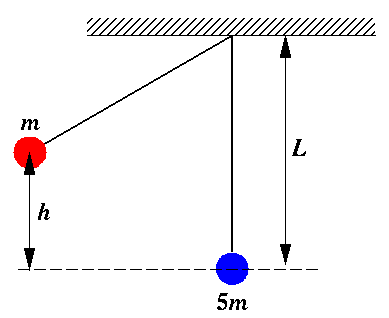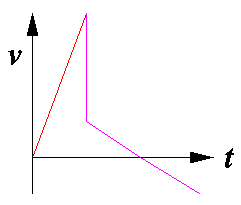## Ballistic Pendulum

Directions: Select the best possible answer from the choices A-D. Guessing will not (on average) reduce your score, but if you have no idea how to work a problem, leave it blank and I'll give you the expected ¼ point.A red sphere (of mass m) and a blue sphere (of mass 5m) are attached to the ceiling by massless strings of identical length forming twin pendulums of length L. The red sphere is drawn to the left so that its center of mass has been raised a distance h and is then released. As the red sphere moves back through equilibrium it hits and adheres to the blue sphere. The combined system swings to the right, eventually reaching some maximum height before swinging back to equilibrium.

1. How much work did gravity do on the red sphere when it was moved from equilibrium to its release point (as displayed above).
1. mgh
2. -mgh
3. ½ mgh
4. cannot be determined
```A B C D
```

2. What was the speed of the red sphere just before its collision?
1. (2gh)½
2. (gh)½
3. g(L2-h2)½
4. ½ gh2
```A B C D
```

3. What was the speed of the red sphere just after its collision?
1. (1/2) its speed before the collision
2. (1/5) its speed before the collision
3. (2/5) its speed before the collision
4. (1/6) its speed before the collision
```A B C D
```

4. If the collision were elastic rather than inelastic how would the post-elastic-collision speeds compare to the post-inelastic-collision speed?
1. The red sphere would be moving faster, the blue slower.
2. The red sphere would be moving slower, the blue faster.
3. Both red and blue would be moving faster than the post-inelastic-collision speed.
4. Neither red and blue would be moving faster than the post-inelastic-collision speed.
```A B C D
```

5. In this problem:
1. momentum and energy are always conserved.
2. potential energy is converted into kinetic energy, but their total is conserved.
3. angular momentum is conserved since the string exerts no torque.
4. momentum is conserved only during the collision and mechanical energy is conserved at all times except during the collision.
```A B C D
```

6. The maximum height reached by the combined spheres is:
1. (1/5) h
2. (1/6) h
3. (1/10) h
4. (1/36) h
```A B C D
```

7. The maximum height reached by the combined spheres would be most increased by:
1. doubling the initial height (h)
2. halving the mass of the blue ball (to 2.5m)
3. doubling the mass of the red ball (to 2m)
4. either B or C
```A B C D
```

8. Which graph best represents the horizontal velocity of the red sphere as a function of time?

ABCD```A B C D
```

9. Aside from the moment of the collision, the acceleration of the red sphere is largest:
1. at the time of release
2. just before the collision
3. just after the collision
4. when the combined spheres reach their maximum height
```A B C D
```